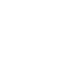# SAT Question of the Day (ACT too!): Oct. 1, 2013

If you are reading this in an email you received from me, do not click the link to sat.collegeboard.org below. Use the link to my website that is farther down on the email. If you are seeing this in my blog, do the SAT Question of the Day by clicking on this link:

http://sat.collegeboard.org/practice/sat-question-of-the-day?questionId=20131001&oq=1 (This link takes you to today’s question. If you use my archive, you will see the question related to my SAT explanation for that date.)

The answer is A.  The key to all circle questions is the radius.  (See Video #7.)  Sometimes the test writer gives it to you directly and sometimes you have to derive it from the area, circumference, or diameter.  In this case, they tell you it is r, so no calculation is required.  The arc AXB is going to be a portion of the circumference.  If you don’t remember how to calculate a circumference, just refer to the cheat sheet (math reference information) at the beginning of the SAT math section.  The circumference is 2Πr.  The equilateral triangle creates three equal arcs so simply divide by 3.  Answer A.

What if you can’t do the geometry?  Don’t despair.  Let’s make an educated guess.  Here are a couple of ways to analyze the answers so that you can make an educated guess.  These techniques work very well on the ACT and less well on the SAT, but let’s see what we can do.  First, you can eliminate D and E quite easily.  The radius squared (r2) is related to the area of a circle, not the circumference; so, eliminate them.  Or you could rule them out by using my “eliminate the oddball” (or “pick common elements”) strategy–three r‘s are not squared and two r‘s are squared.  Because r is more common among the answers than r-squared, get rid of the r-squareds and pick the common element r.  Those are two reasons to get rid of D and E.

Now, what about answers A, B, and C?  Using the “eliminate the oddball/pick common element” technique, you can throw out C because it is the only answer with 2 in the denominator and pick one of the other answers because they both have a 3 in common in their denominators.  That sure makes C an “oddball.”  Now we are down to A and B.  A has a 2 in the numerator as does E.  So, there are two answers with 2 in common in the numerator.  1, 3, and 4 appear only once in the numerators; so, they are “oddballs.”  Guess Answer A.  You happen to get lucky and get the right answer to this question.

I’m NOT suggesting you go through the test and simply guess without doing any math!  I am just pointing out that you can raise your score by analyzing answers as a strategy when you are stuck on the math.  “Eliminating the oddball” or “pick common elements” simply makes you a better guesser and typically works on the ACT much better than the SAT.  Because there’s no guessing penalty on the ACT, I always suggest using the “oddball” strategy rather than random guessing.  Play the game and raise your score!

Of course, there will be answers that don’t have common elements at all.  For example, if the answers are all just numbers like 3, 7, 11, 14, and 19, you can’t do this.  There aren’t any common elements as there are in today’s SAT Question of the Day example.

Let’s see what the ACT folks have for us today.

The answer is B.  The slope-intercept equation is a common math concept that shows up on both the SAT and ACT.  (See Video #8.)  These questions are easy to recognize because you’ll see the word “slope” in the question and then an equation that relates y and x as you see in this question.  You simply have to divide both sides of the equation by 5 an you’ll get -3/5 for the coefficient for x which tells you the slope.

Let’s see how “eliminate the oddball” works on this question.  There are two negative and three positive answers; so, you should pick a positive.  Oops!  There are 3 integers and and two fractions; so, you should eliminate the fractions.  Oops!  See, I told you it doesn’t always work and you should try to do the math first.  The strategy isn’t a guarantee to get the right answer; it just changes your odds from 1 in 5 to something better than that.

I’m actually glad the strategy didn’t work on this question.  If it had, some of you would put too much faith in the technique.  Keep in mind it is a process you can use when you have no idea how to do the math  and want to make a better guess.

Happy October!  If you are a baseball fan, it’s a great time of the year.  Enjoy.

The SAT & ACT Wizard